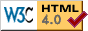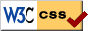## KeyPairGenerators

[TODO: ECDH, ECDSA, RW]

 DH KeyPairGenerator
Description:
[[need more detail]]
References:
• [Inf] Whitfield Diffie et al,
"New Directions in Cryptography,"
IEEE Transactions on Information Theory, vol. IT-22, No. 6, pp. 644-654. November 1976.
• [An] Paul C. van Oorschot, Michael J. Wiener,
"On Diffie-Hellman Key Agreement with Short Exponents,"
Advances in Cryptology - EuroCrypt '96. Springer-Verlag.
PublicKey parameters:
• `BigInteger p` [read] - the prime modulus, p
• `BigInteger g` [read] - the base, g
• `BigInteger y` [read] - the public value, gx mod p
• `BigInteger order` [optional, read] - the order of the subgroup generated by g (see comment below)
PrivateKey parameters:
• `BigInteger p` [read] - the prime, p
• `BigInteger g` [read] - the base, g
• `BigInteger x` [read] - the private value, x
• `BigInteger y` [read] - the public value, gx mod p
• `BigInteger order` [optional, read] - the order of the subgroup generated by g (see comment below)
Comment:
If the `order` parameter is present in the public key, then encryption or key agreement will be done in the subgroup of the given order generated by g. I.e. the random value k used to calculate gk will be a "short exponent" in the range [1, order]. Implementations MAY exclude 1 and order from this range, if the definition of the encryption or key agreement algorithm allows it (since they occur with negligable probability, this will not affect security).

`order` MAY be present in the private key, but is not needed for private key operations (since the private exponent x determines its own length).

• p should typically be at least 1024 bits in length.
• `order`, if present, should be at least 2160, and preferably prime.

 DSA KeyPairGenerator
Description:
The key pair generation algorithm described in NIST FIPS PUB 186 for DSA.
Alias:
"1.2.840.10040.4.3"
Length:
The length, in bits, of the modulus p. This can be any integer that is a multiple of 8, greater than or equal to 512.
References:
PublicKey parameters:
• `BigInteger p` [read] - the prime modulus, p
• `BigInteger q` [read] - a prime factor of p-1, q
• `BigInteger g` [read] - h(p-1)/q mod p, where h is less than p-1 and g > 1
• `BigInteger y` [read] - gx mod p
PrivateKey parameters:
• `BigInteger p` [read] - the prime, p
• `BigInteger q` [read] - a prime factor of p-1, q
• `BigInteger g` [read] - h(p-1)/q mod p, where h is less than p-1 and g > 1
• `BigInteger x` [read] - the private value, < q
• `BigInteger y` [read] - gx mod p
Parameter defaults:
[see parameter defaults for the DSA AlgorithmParameterGenerator]

 ECDH KeyPairGenerator
Description:
[[need more detail]]
References:
• [Def] X9.62-199x (draft), Public Key Cryptography For The Financial Services Industry: The Elliptic Curve Digital Signature Algorithm (ECDSA).
• [Inf] L. Bassham, D. Johnson, W. Polk,
Internet X.509 Public Key Infrastructure: Representation of Elliptic Curve Digital Signature Algorithm (ECDSA) Keys and Signatures in Internet X.509 Public Key Infrastructure Certificates,
October 1999 Internet draft.
http://www.pca.dfn.de/dfnpca/drafts/draft-ietf-pkix-ipki-ecdsa-02.txt
PublicKey parameters:
• `ECParameters params` [read] - the curve parameters and base point
• `ECPoint P` [read] - the public point
PrivateKey parameters:
• `ECParameters params` [read] - the curve parameters and base point
• `ECPoint P` [read] - the public point
• `BigInteger x` [read] - the private value, x
Patent status:
• Certicom has patents pending on several techniques for efficient implementation of elliptic curve arithmetic (these mainly apply to hardware implementations using an Optimal Normal Basis).
• RSA Data Security, Inc. has a patent [[pending??]] on a technique for conversion between polynomial and Optimal Normal Basis representations of elements of GF(2m).

 ECDSA KeyPairGenerator
Description:
The format of a BER or DER-encoded ECParameters object, as defined in X9.62. The ASN.1 syntax of ECParameters is given in the description of ECDH/ASN.1.

Output MUST be encoded as DER.

Aliases:
"1.2.840.10045.2.1"
References:
• [Def] X9.62-199x (draft), Public Key Cryptography For The Financial Services Industry: The Elliptic Curve Digital Signature Algorithm (ECDSA).
• [Inf] L. Bassham, D. Johnson, W. Polk,
Internet X.509 Public Key Infrastructure: Representation of Elliptic Curve Digital Signature Algorithm (ECDSA) Keys and Signatures in Internet X.509 Public Key Infrastructure Certificates,
October 1999 Internet draft.
http://www.pca.dfn.de/dfnpca/drafts/draft-ietf-pkix-ipki-ecdsa-02.txt
Patent status:
[see general elliptic curve patents]

 ElgamalSig KeyPairGenerator
Description:
[[need more detail]]
References:
• [Def] Taher Elgamal,
"A Public-Key Cryptosystem and a Signature Scheme Based on Discrete Logarithms,"
IEEE Transactions on Information Theory, v. IT-31, n. 4, 1985, pp. 469-472, or
Advances in Cryptology - CRYPTO '84, pp. 10-18, Springer-Verlag, 1985.
• [Inf] D. Bleichenbacher,
"Generating ElGamal signatures without knowing the secret key,"
Advances in Cryptology - EUROCRYPT '96 (corrected version), Volume 1070 of Lecture Notes in Computer Science, pp. 10-18. Springer Verlag, 1996.
ftp://ftp.inf.ethz.ch/pub/publications/papers/ti/isc/ElGamal.ps
PublicKey parameters:
• `BigInteger p` [read] - the prime modulus, p
• `BigInteger g` [read] - the base, g
• `BigInteger y` [read] - the public value, gx mod p
PrivateKey parameters:
• `BigInteger p` [read] - the prime, p
• `BigInteger g` [read] - the base, g
• `BigInteger x` [read] - the private value, x
• `BigInteger y` [read] - the public value, gx mod p
Comment:
Use of "short exponents" is not supported.
• If the key parameters are not given explicitly, then p and g SHOULD be chosen so that p is a safe prime, i.e. such that (p-1)/2 is prime, and g generates the order (p-1)/2 subgroup of GF(p).
• The paper by Bleichenbacher referenced above shows that if g has only small prime factors, and if g divides the order of the group it generates, then signatures can be forged. Implementations MUST NOT generate key pairs that would allow this attack.

 RSA KeyPairGenerator
Aliases:
"1.2.840.113549.1.1.1", "2.5.8.1.1"
References:
• [Inf] PKCS #1: RSA Encryption Standard,
An RSA Laboratories Technical Note, Version 1.5. Revised November 1, 1993.
ftp://ftp.rsa.com/pub/pkcs/ascii/pkcs-1.asc
• [Inf] Bruce Schneier,
"Section 19.3 RSA,"
Applied Cryptography, Second Edition, John Wiley & Sons, 1996.
• [An] R. Rivest, R. Silverman,
"Are 'Strong' Primes Needed for RSA?"
December 1, 1998 (also RSA Laboratories tech note, August 1, 2000).
http://www.rsalabs.com/technotes/
PublicKey parameters:
• `BigInteger modulus` [read] - the modulus, n
• `BigInteger publicExponent` [read] - the public exponent, e
PrivateKey parameters:
Either, or both, of the following two sets of parameters:
• `BigInteger modulus` [read] - the modulus, n
• `BigInteger privateExponent` [read] - the private exponent, d
and
• `BigInteger primeP` [read] - one of the prime factors of the modulus, p
• `BigInteger primeQ` [read] - the other prime factor of the modulus, q
• `BigInteger primeExponentP` [read] - d mod (p-1)
• `BigInteger primeExponentQ` [read] - d mod (q-1)
• `BigInteger crtCoefficient` [read] - q-1 mod p

It does not matter which of p and q is larger.Author: David Hopwood Current maintainer: David Hopwood Copyright © 1995-2001 The Cryptix Foundation Limitedand David Hopwood. All rights reserved. Cryptix is a trademark of The Cryptix Foundation Limited.